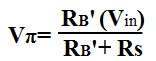﻿ How to Calculate gmVπ of a Transistor

# How to Calculate gmVπ of a Transistor?GmVπ, the current gain of a transistor, is an important calculation because it determines the transistor's chief characteristic, which is its gain.

GmVπ can only be calculated by doing both AC and DC analysis of a transistor circuit.

To find gm of the transistor, DC analysis must be done on the transistor circuit. Once all DC values are computed, gm can be calculated by the formula:Next is to find of the transistor circuit. In order to find Vπ, AC analysis must be done on the transistor circuit. Once all AC values are computed, Vπ can be calculated by the formula: